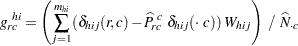### Row and Column Proportions

PROC SURVEYFREQ computes the estimate of the row proportion for table cell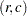as the ratio of the estimated total for the table cell to the estimated total for row,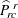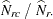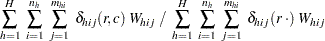Similarly, PROC SURVEYFREQ estimates the column proportion for table cellas the ratio of the estimated total for the table cell to the estimated total for column,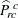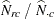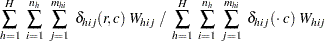If you request BRR variance estimation (VARMETHOD=BRR), PROC SURVEYFREQ estimates the variances of the row and column proportions as described in the section Balanced Repeated Replication (BRR). If you request jackknife variance estimation (VARMETHOD=JACKKNIFE), the procedure estimates the variances as described in the section The Jackknife Method.

If you do not specify the VARMETHOD= option or a REPWEIGHTS statement, the default variance estimation method is Taylor series (VARMETHOD=TAYLOR). By using Taylor series linearization, the variance of the row proportion estimate can be expressed aswhere if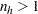,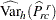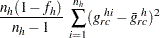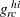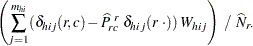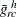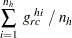and if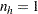,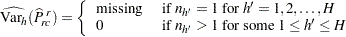The standard error of the row proportion is computed as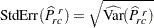The Taylor series variance estimate for the column proportion is computed as described previously for the row proportion, but with